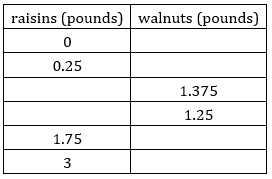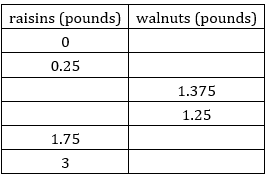# A.2.12.2 Trail Mix

You will now examine the same situation involving two quantities (raisins and walnuts), but there are now two constraints (cost and weight). Here is a situation you saw earlier: Diego bought some raisins and walnuts to make trail mix. Raisins cost \$4 a pound and walnuts cost \$8 a pound. Diego spent \$15 on both ingredients. Write an equation to represent this constraint. Let x be the pounds of raisins and y be the pounds of walnuts.

Graph your equation from above on the graph below.

Complete the table with the amount of one ingredient Diego could have bought given the other.Here is a new piece of information: Diego bought a total of 2 pounds of raisins and walnuts combined. Write an equation to represent this new constraint. Use the same variables as before: let x be the pounds of raisins and y be the pounds of walnuts.

Graph your equation from above on the graph below.

Complete the table with the amount of one ingredient Diego could have bought given the other.Diego spent \$15 and bought exactly 2 pounds of raisins and walnuts. How many pounds of each did he buy?